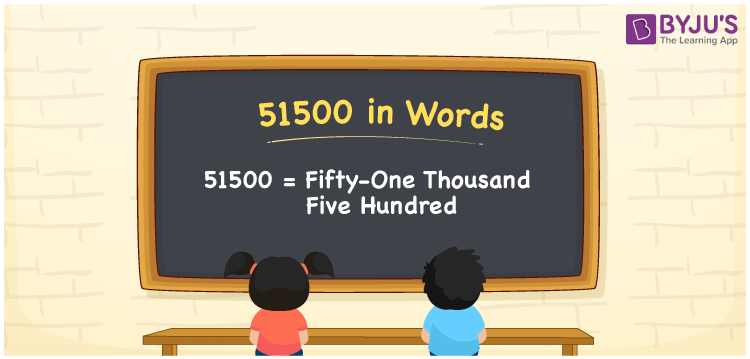# 51500 in Words

51500 in words is written as Fifty-One Thousand Five Hundred. If you saved Rs. 51500 in three months, you can say, “I saved Rupees Fifty One Thousand Five Hundred in three months”. It is vital to create a place value chart to write the given number in word form. We know that 51500 is a cardinal number since it shows the value of something. In this article, we will learn the method of writing the number 51500 in word form.

 51500 in Words Fifty-One Thousand Five Hundred Fifty-One Thousand Five Hundred in numerical form 51500

## 51500 in English Words

Generally, numbers in words are formed by using the English alphabet. Therefore, 51500 is written in words as Fifty-One Thousand Five Hundred.## How to Write 51500 in Words?

We use a place value chart to convert the numbers in word form. A place value chart shows the positions of each digit in a number. Let us prepare a place value chart of up to five digits as 51500 has five digits. The place value chart for the number 51500 is shown below:

 Ten-Thousands Thousands Hundreds Tens Ones 5 1 5 0 0

Thus, we can write the expanded form as:

5 x Ten Thousand + 1 x Thousand + 5 x Hundred + 0 x Ten + 0 x One

= 5 x 10000 + 1 x 1000 + 5 x 100 + 0 x 10 + 0 x 1

= 50000 + 1000 + 500 + 0 + 0

= 50000 + 1000 + 500

= 51500

= Fifty-One Thousand Five Hundred

Therefore, 51500 in words is written as Fifty-One Thousand Five Hundred.

Interesting way of writing 51500 in words

5 = Five

51 = Fifty-One

515 = Five Hundred and Fifteen

5150 = Five Thousand One Fifty

51500 = Fifty-One Thousand Five Hundred

Thus, the word form of the number 51500 is Fifty-One Thousand Five Hundred.

51500 is a natural number that is succeeded by 51499 and preceded by 51501

• 51500 in words – Fifty-One Thousand Five Hundred
• Is 51500 an odd number? – No
• Is 51500 an even number? – Yes
• Is 51500 a perfect square number? – No
• Is 51500 a perfect cube number? – No
• Is 51500 a prime number? – No
• Is 51500 a composite number? – Yes

## Frequently Asked Questions on 51500 in Words

### How do you write 51500 in words?

51500 in words is given by Fifty-One Thousand Five Hundred.

### What is the place value of 1 in 51500?

The place value of 1 in 51500 is Thousands.

### Write Fifty-One Thousand Five Hundred in numbers.

Fifty-One Thousand Five Hundred in numbers is 51500.